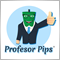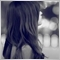55

```int totalorder(int m)
{
int total=0;
for(int i=0; i<OrdersTotal(); i++)
{
if(OrderSymbol()!=Symbol() || OrderMagicNumber()!=MagicNumber || OrderType()!= m) continue;
total++;
}
return(total);
}
double open(int m)
{
for (i = 0; i < OrdersTotal(); i++)
{
if (OrderSymbol() != Symbol() || OrderMagicNumber() != Magic ||OrderType()!=m) continue;
dlot=OrderOpenPrice();
}
return(dlot);
}

if(totalorder(0)==0  && totalorder(1)==0 && Sell_only && signal==2) //sell 1
{if(OrderSend(Symbol(),OP_SELL,NR(Lots),Bid,3,sls, tps,Nama_EA,Magic,0,Red))}

bidi = open(1) + 20pips;

if( Bid >= bidi && totalorder(1)>0 && totalorder(0)< Max_order) //sell 1+n
{if(OrderSend(Symbol(),OP_SELL,xlot(1),Bid,3,sls, tps,Nama_EA,Magic,0,Red))} ```

I want to store and count the virtual trade but i have no idea to start since the above code need to make the trade. base on code above how do i twist to make it store a count variable without make it open trade?268

Hello, you can count how many times magic number was appears or write other EA to counting, maybe work in this case. Regards Greg245

Okay, let's analyze the solution for you!

The first: We must know the root of the problem.

- How can we know the price (OLHC) of the symbol, and how many orders or how much their floating F/L are we having?

- The answer is: Database. Yes, what we are seeing in MT4/MT5 is the real-time database of the Broker. And, the Broker retrieves the database from the higher level: Market.

- So, we know the root of the problem now, right? It's database.

Next: Build the the way to implement what you want to do as a diagram.

- The simplest database machine is variable or text file (or something like it). If you are planning to use an ORM, that's great. Now I'm talking about the variable or text file.

- Case 1: If you use your EA to trade one pair only, just simply use a global variable & implement a function to increase or decrease its value.

- Case 2: If you use your EA to trade more than one pair in one chart window only, just simple use an two-dimension array global variable & implement a function with argument(s) to increase / decrease each variable's value.

- Case 3: If you use your EA to trade more than one pair, but in multi chart window (trade EU in EU window, GU in GU window, ...), now the problem is more complex; it must be a cross-border variable, right? The solution for this case is: store order number of each symbol in a separate text file, and you have to implement two necessary functions, the first is for increase/decrease order number of each symbol purpose, one is for count all text file to know the total order number purpose. Why do we have to use multiple text file? Because, using the text file to store value as a database machine is different from one ORM (such as MySQL, SQLSERVER, ...), we can't modify one text file by multiple programs @ the same time but have no problem.

The last: Sample code as flowchart (I have never done with text file on MQL, so use the global variable only):

Case 1:

Have one global variable named EURUSD_OrderSum = 0;

Have one function increaseDecrease(int number) {

return EURUSD_OrderSum + n;

}

increaseDecrease(1);

}

}

if(okay to close){

increaseDecrease(-1);

}

}

Case 2: Nearly same as case 1, have a bit difference.

You'll need to have one two-dimension array global variable: int orderSum = {{0}, {0}}; // The first index is various, it depends on how many pairs the EA trades.

And the increase/decrease function must have one more argument increaseDecrease(int resource, int number). The resource is the index of the array when the program calls.

Case 3: I have never done with text file on MQL before, so you have to learn yourself :D55

Greg Pawlak:
Hello, you can count how many times magic number was appears or write other EA to counting, maybe work in this case. Regards Greg

can you provide some example?

the main idea is like

Virtual- (how do i count the virtual trade? i dont want to execute them..i just want the count number )55

Giang Nguyen:

Okay, let's analyze the solution for you!

The first: We must know the root of the problem.

- How can we know the price (OLHC) of the symbol, and how many orders or how much their floating F/L are we having?

- The answer is: Database. Yes, what we are seeing in MT4/MT5 is the real-time database of the Broker. And, the Broker retrieves the database from the higher level: Market.

- So, we know the root of the problem now, right? It's database.

Next: Build the the way to implement what you want to do as a diagram.

- The simplest database machine is variable or text file (or something like it). If you are planning to use an ORM, that's great. Now I'm talking about the variable or text file.

- Case 1: If you use your EA to trade one pair only, just simply use a global variable & implement a function to increase or decrease its value.

- Case 2: If you use your EA to trade more than one pair in one chart window only, just simple use an two-dimension array global variable & implement a function with argument(s) to increase / decrease each variable's value.

- Case 3: If you use your EA to trade more than one pair, but in multi chart window (trade EU in EU window, GU in GU window, ...), now the problem is more complex; it must be a cross-border variable, right? The solution for this case is: store order number of each symbol in a separate text file, and you have to implement two necessary functions, the first is for increase/decrease order number of each symbol purpose, one is for count all text file to know the total order number purpose. Why do we have to use multiple text file? Because, using the text file to store value as a database machine is different from one ORM (such as MySQL, SQLSERVER, ...), we can't modify one text file by multiple programs @ the same time but have no problem.

The last: Sample code as flowchart (I have never done with text file on MQL, so use the global variable only):

Case 1:

Have one global variable named EURUSD_OrderSum = 0;

Have one function increaseDecrease(int number) {

return EURUSD_OrderSum + n;

}

increaseDecrease(1);

}

}

if(okay to close){

increaseDecrease(-1);

}

}

Case 2: Nearly same as case 1, have a bit difference.

You'll need to have one two-dimension array global variable: int orderSum = {{0}, {0}}; // The first index is various, it depends on how many pairs the EA trades.

And the increase/decrease function must have one more argument increaseDecrease(int resource, int number). The resource is the index of the array when the program calls.

Case 3: I have never done with text file on MQL before, so you have to learn yourself :D

err man..thanks for the tips but this step is beyond upper my imagination lol.268

can you provide some example?

the main idea is like

Virtual- (how do i count the virtual trade? i dont want to execute them..i just want the count number )